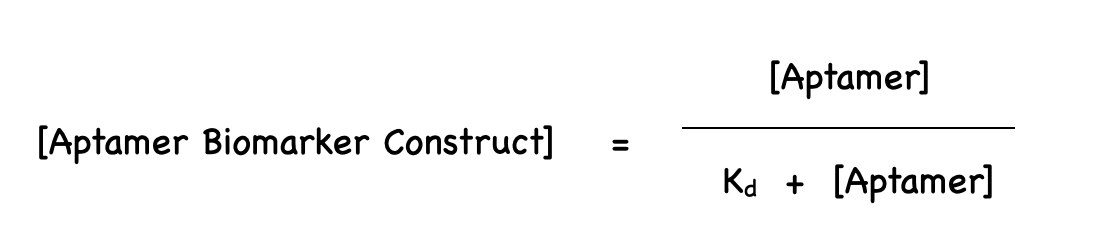# Team:ASIJ Tokyo/Math Model

​​

## Equation Derivation

###### Our equation was derived from the rate equation commonly used in chemistry used to model the interaction between two elements: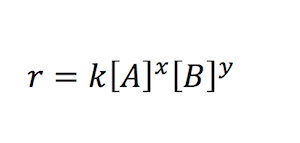###### For our experiment, we defined “A” as our aptamer, “B” as our biomarker and “AB” as our aptamer biomarker construct to model the interaction between aptamer and biomarker:###### (Derivatives, or rates, are noted by a · (dot), and concentrations are represented by [] (brackets).)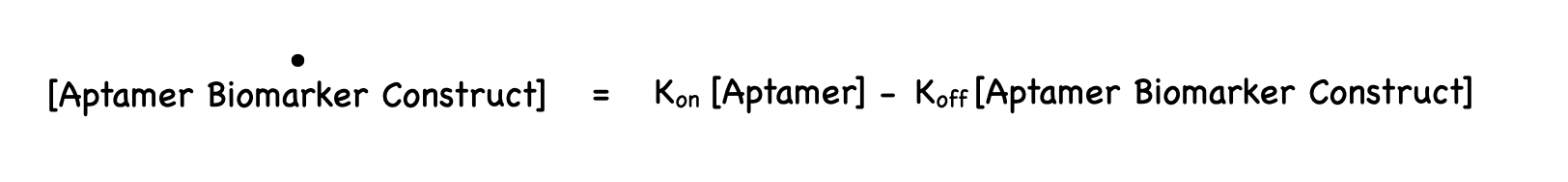###### Likewise, the rate of biomarker interaction at equilibrium (when the rate is 0) can be modelled in the following manner: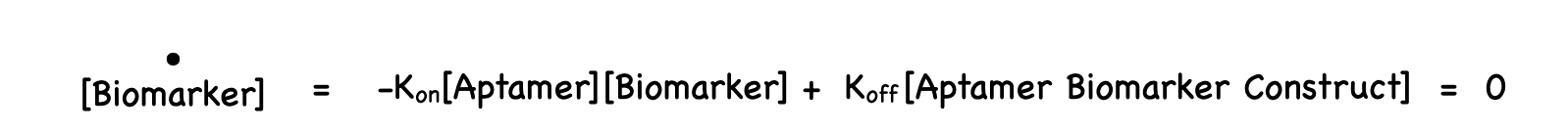###### Thus, at equilibrium, the interaction can be written as the following by applying the above equation after adding the aptamer and biomarker concentrations to the other side: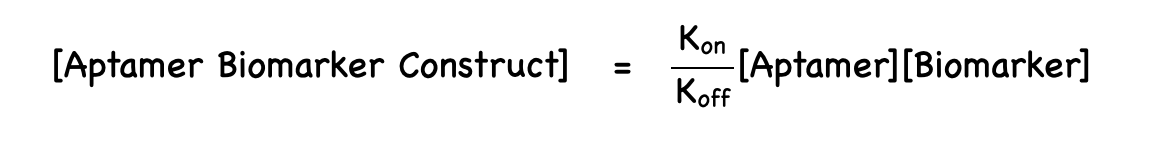###### Using algebra and simplification: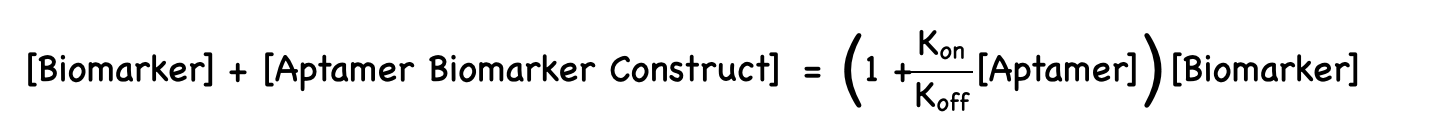###### Now, we use the fact that the ratio of aptamer biomarker constructs is given by the amount of aptamer biomarkers construct divided by the total number of aptamers (including those currently binding). Collated with the expression Koff /Kon (which is equal to [A][B]/[AB] by definition) this gives: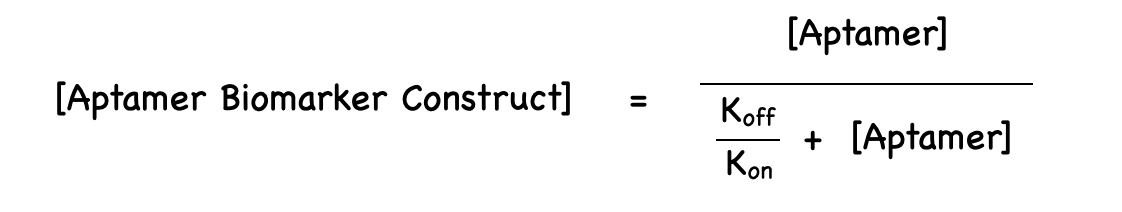###### Thus, the final equation to model the proportion of interaction of aptamer and biomarker forming the aptamer and biomarker construct can be written as: